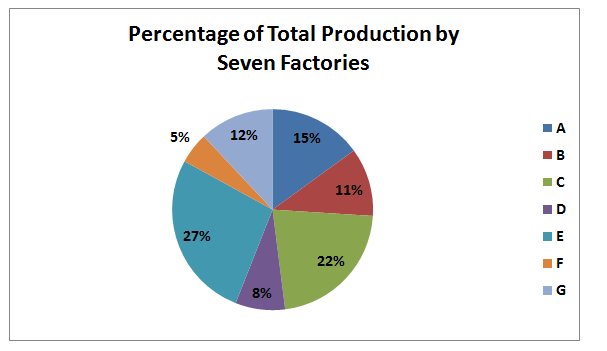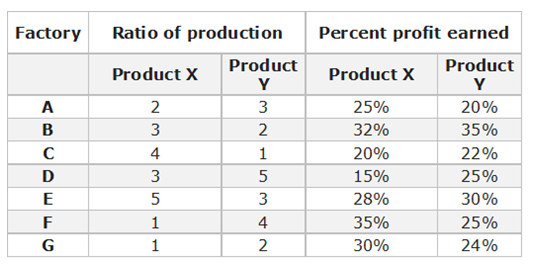# “20-20” Quantitative Aptitude | Crack SBI Clerk 2018 Day-35

Dear Friends, SBI Clerk 2018 Notification has been released we hope you all have started your preparation. Here we have started New Series of Practice Materials specially for SBI Clerk 2018. Aspirants those who are preparing for the exams can use this “20-20” Quantitative Aptitude Questions.

## Daily Practice Test Schedule | Good Luck

 Topic Daily Publishing Time Daily News Papers & Editorials 8.00 AM Current Affairs Quiz 9.00 AM Logical Reasoning 10.00 AM Quantitative Aptitude “20-20” 11.00 AM Vocabulary (Based on The Hindu) 12.00 PM Static GK Quiz 1.00 PM English Language “20-20” 2.00 PM Banking Awareness Quiz 3.00 PM Reasoning Puzzles & Seating 4.00 PM Daily Current Affairs Updates 5.00 PM Data Interpretation / Application Sums (Topic Wise) 6.00 PM Reasoning Ability “20-20” 7.00 PM English Language (New Pattern Questions) 8.00 PM General / Financial Awareness Quiz 9.00 PM

[WpProQuiz 1403]

Click “Start Quiz” to attend these Questions and view Explanation

### Click Here for SBI Clerk Prelims and Mains 2018 – Full Length Mock Test

Directions (Q. 1-5): What will come in place of question mark (?) in the following questions?

1. (8/5) of 404 + 98% of 820 + (5/4) ÷ (9/63) =?
1. 1458.75
2. 1326.50
3. 1234.25
4. 1678.50
5. None of these
1. 0.8% of 3072 + 12% of 785 =?
1. 128.546
2. 132.668
3. 118.776
4. 146.782
5. None of these
1. 2162 ÷ 46 × 23 + 287 -189 =?
1. 1239
2. 1568
3. 1743
4. 1179
5. None of these
1. ? % of (400 + (16 ÷ 0.2) ÷ 0.05) =1700
1. 85
2. 78
3. 92
4. 68
5. None of these
1. 8195 ÷ 745 + ?/12 =7847
1. 78045
2. 94032
3. 86512
4. 56056
5. None of these

Directions (Q. 6-10): What should come in place of question mark (?) in the following number series:

1. 936, 156, 31.2, ? , 2.6, 1.3
1. 7.8
2. 15.6
3. 8.6
4. 7.2
5. None of these
1. 9, 5, 6, 10.5, 23, ?
1. 78
2. 60
3. 88
4. 120
5. None of these
1. 7, 20, 46, 98, 202, ?
1. 350
2. 560
3. 410
4. 670
5. None of these
1. 10, 11, 26, 87, ? , 1845
1. 638
2. 568
3. 734
4. 364
5. None of these
1. 16, 121, 841, 5041, ?
1. 25201
2. 32407
3. 15607
4. 38301
5. None of these
1. The average monthly income of a family of 6 members was 21600. One of the daughters in the family got married and left home, so the average monthly income of family comes down to 18200. What is the monthly income of married daughter?
1. 23400
2. 38600
3. 12900
4. 28900
5. None of these
1. 5 years ago, the ratio of ages of Abi and Pari was 5:3. Neela is 5 years younger than Abi and 5 years older than Pari. What is Neela’s present age?
1. 20 years
2. 22 years
3. 25 years
4. 30 years
5. 28 years
1. The difference between the S.I and C.I on a certain sum at 5% per annum for 2 years is RS. 105. Find the sum?
1. 36000
2. 28000
3. 46000
4. 42000
5. None of these
1. Two trains 155m and 175 m long respectively are running in the same direction at the speed of 81 km/hr and 45 km/hr respectively. In how much time will the first train take to cross the second one?
1. 16 sec
2. 27 sec
3. 42 sec
4. 33 sec
5. None of these
1. A boat can cover 9.6 km upstream in 24 minutes. If the speed of the current is ¼ of the boat in still water, then how much distance can the boat cover downstream in 36 minutes?
1. 24 km
2. 36 km
3. 28 km
4. 42 km
5. None of these

Directions (Q. 16 – 20): Study the following charts and answer the following questions

Cost of total production (both products together) by seven factories = 25 CroreRatio of production between product X and Y and the percent profit earned by the two product1. Cost of production of product X by factory F is what percent of the cost of production of product Y by factory D?
1. 10%
2. 15%
3. 16.67%
4. 33.33%
5. None of these
1. What is the total profit earned by factory G for products X and Y together?
1. 78 lakh
2. 1.62 cr
3. 7.8 lakh
4. 68 lakh
5. None of these
1. What is the ratio of the cost of production of product X by factory A to the cost of production of product X by factory D?
1. 1:2
2. 1:3
3. 3:1
4. 2:1
5. None of these
1. What is the amount of profit earned by factory D on product Y? (approx.)
1. 21 lakh
2. 28 lakh
3. 31 lakh
4. 35 lakh
5. None of these
1. What is the cost of production of product X by factory A, B and D together?
1. 2.90cr
2. 3.90cr
3. 4.10cr
4. 4.40cr
5. None of these

Directions (Q. 1-5):

(8/5) × 404 + (98/100) × 820 + (5/4) ÷ (9/63) =x

X= 646.4 + 803.6 + 8.75

X= 1458.75

0.8% of 3072 + 12% of 785 =x

X= (8/1000) × 3072 + (12/100) × 785

X= 24.576 + 94.2

X= 118.776

2162 ÷ 46 × 23 + 287 -189 =x

X= 1081 +287 -189

X= 1179

x % of (400 + 16 ÷ 0.2 ÷ 0.05) =1700

(x/100) × (400 + ((160/2) × (100/5)) =1700

(x/100) × (400 + 1600) =1700

(x/100) × 2000 = 1700

20x = 1700

X= 85

8195/745 + x/12 =7847

11 + x/12 =7847

x/12 = 7836

x= 94032

Directions (Q. 6-10):

The pattern is, ÷ 6, ÷ 5, ÷ 4, ÷ 3, ÷ 2

The pattern is, *0.5 + 0.5, *1 +1, *1.5 +1.5, *2 +2, *2.5 +2.5

The pattern is, +(13*1), +(13*2), +(13*4), +(13*8), +(13*16)

The pattern is, *1+12, *2+22, *3+32, *4+42, *5+52

The pattern is, *8 – 7, *7 -6, *6 -5, *5 -4,..

The average monthly income of a family of 6 members was 21600,

Total monthly income of a family of 6 members= 21600*6= 129600

One of the daughters got married and left the home, now the average = 18200

Total monthly income of a family of 5 members = 18200*5 = 91000

Monthly income of married daughter= 129600 – 91000 = 38600

5 years ago, the ratio of ages of Abi and Pari = 5:3 (let us assume the age of abi and pari = 5x, 3x)

Neela = Abi – 5

Neela = Pari + 5

Abi – 5 = Pari + 5

(5x + 5) – 5 = (3x + 5) + 5

2x= 10=> x= 5

Abi= 25+5 = 30, Pari= 15+5 =20

Neela’s Present age= Abi – 5= 25 years

The difference between the S.I and C.I for 2 years,

Diff= sum*(r/100)2

105 = sum*(5/100)2

Sum= 42000

Both the trains are running at the same direction,

So, relative speed= S1 – S2

Distance= Train length1+ Train length2

The first train takes to cross the second one in,

T = D/S

T= (155+175)/((81 – 45)*(5/18))

T= 330/(36*(5/18))

T= 330/10 = 33 sec

The speed of upstream= D/T = 9.6/(24/60) = 24 km/hr

The speed of the current =  ¼ of the boat in still water

Speed of boat : Speed of Current = 4:1 = (x: 4x)

Speed of Upstream = Speed of boat – Speed of Current = 3x

24 = 3x

X= 8

Speed of boat = 32 km/hr, Speed of Current = 8 km/hr

Speed of downstream= Speed of boat + Speed of Current = 5x

Speed of downstream = 40 km/hr

Time = 36 min= (36/60) hr

Distance= S*T = 40*(36/60) = 24 km

Directions (Q. 16 – 20):

For factory F = 25*5/100*1/5 and for factory D = 25*(8/100)*(5/8)

% percent = [25*5/100*1/5]/ [25*8/100*5/8] = 1/5*100 = 20%

For product X cost of production = 25*12/100*1/3 = 1 crore and for product Y it is 2 crore.

Now profit earned on X = 1*130/100 – 1 = 0.3 crore and profit earned on Y = 2*124/100- 2 = 0.48 crore.

Total profit = 0.3 + 0.48 = 0.78 crore or 78 lakh

Ratio = 25*15/100*2/5 : 25*8/100*3/8 = 2:1

(8/100)*25*(5/8) = 1.25 crore

1.25*(125/100) = 1.56 crore, profit = 1.56 – 1.25 = 31 lakh